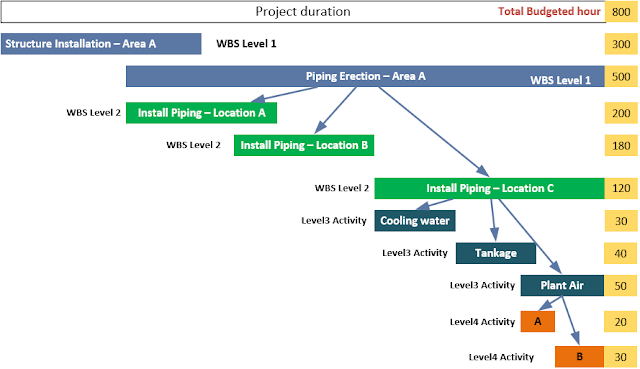## Pages

• Home
• Tutorials

### How to Get Earned Value/Progress of Each Activity to Overall Project

Once you’ve developed planned value data, the next step is to calculate earned value from the lowest activities to track overall project progress. To measure project progress, list the activities (including WBS) with budgeted hours or cost.

See the picture below to understand how you can track the overall progress of the various disciplines – piping and structure.The simple technique to evaluate overall project progress is to find out the progress of lower activities to higher activities.

=> The progress of Plant Air work – Level 3
To illustrate this to get earned progress of Plant Air, calculate the progress of lower activities - Activity A and Activity B. So, follow the steps below:
(1) Check physical progress
Imagine Activity A has two steps – Erecting the pipe and Bolt up/Joining. And, the project team has agreed to claim 50% for erecting the pipe and another 50% for bolt-up/joining. So, when erecting piping work is completed 100% of quantity, then the physical progress is 50% x 100% = 0.5 or 50%.
(1) Calculate earned hour of Activity A = 50% physical progress x 20 hours of budgeted hours = 10 earned hours

(2) Figure out earned hour of Activity B = 0% physical progress x 30 hours of budgeted hours = 0 earned hour
Then, the earned value (hour) of Plant Air piping scope is (2) + (3) = 10 hours.
You can now find out earned progress: Earned hour/Total Budget hour = 10/50 = 20%

=> The progress of Tankage and Cooling water – Level 3: use the same method done for Plant Air to get the earned hours

=> The progress of Install Piping Location – C – Level 2
1) Check the earned hour of each level 3 activities: for example - Plant Air (10 hrs), Tankage (20 hrs) and Cooling water ( 0 hrs)

2) Sum up the earned hours - 10 hrs + 20 hrs + 0 = 30 hrs

3) Check the total budgeted hour of level 2 activity - Install Piping Location – C: 120 hours

4) Calculate earned progress = > 30 / 120 = 25%
=> The progress of Install Piping – Area A – Level 1

1) Check the earned hour of each level 2 activities: for example - Install Piping Location - A (200 hrs), Install Piping Location – B (80 hrs) and Install Piping Location - C ( 30 hrs)

2) Sum up the earned hours - 200 hrs + 80 hrs + 30 = 310 hrs

3) Check the total budgeted hour of level 1 activity - Install Piping – Area A: 500 hours

4) Calculate earned progress = > 310 / 500 = 62%

=> The progress of the overall project (multi-disciplines) – Level 0
1) Check the earned hour of each level 1 activities: e.g., Install Piping – Area A (310 hrs), Install Structure – Area A ( 250 hrs)

2) Sum up the earned hours - 310 hrs + 250 hrs = 560 hrs

3) Check the total budgeted hour – piping and structure scope: 800 hours

4) Calculate earned progress = > 560 / 800 = 70%
That's it! Now you've got progress data. Once the planned and earned progress data are available, plot the S Curve in Excel spreadsheet.

1.1.